Jones Iron Works

# Cases in Cost Management

## Quiz 16 :Jones Iron WorksStudy FlashcardsLooking for Management Homework Help?## Quiz 16 :Jones Iron WorksCalculate the annual DPP per "linear foot" of shelf space for Store #5 as a whole.
Free
Essay

Direct Product Profit (DPP):
Direct product profit is defined as an accounting method for measuring the profitability of the product and the profitability of the shelf space.
By extracting the information: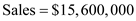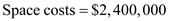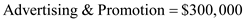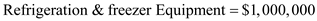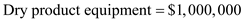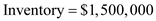Calculate the annual DPP per linear foot for store #5 as a whole:
Methodology:
The DPP approach is designed as follows: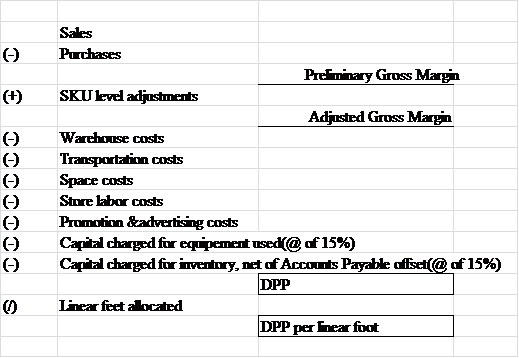Substitute:
Here,
Sale is $15,600,000, space costs is$2,400,000, advertising promotion is $300,000, refrigeration freezer equipment is$1,000,000, dry product equipment is $1,000,000, inventory is$1,500,000, and the rate of capital charged is 15%.
Assumptions:
The warehouse and transportation cost are assumed to be zero as the amount is included in cost of goods sold but the allocation is not given.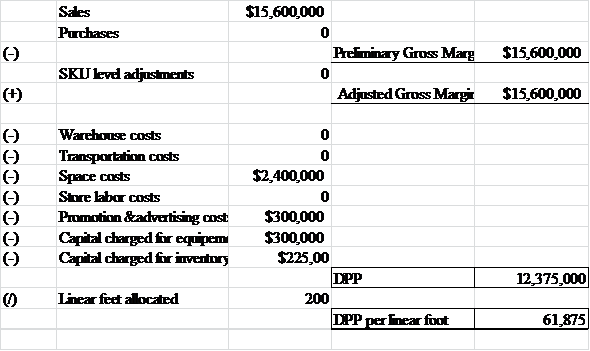Working notes:
Calculation of capital charge for equipment:
Given: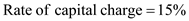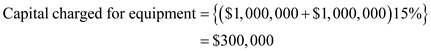Calculation of Capital charge for inventory:
Given: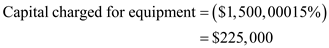Calculate the annual DPP per "linear foot" for Bread for Store #5.
Free
Essay

Direct Product Profit (DPP):
Direct product profit is defined as an accounting method for measuring the profitability of the product and the profitability of the shelf space.
By extracting the information:Dry product equipment $1,000,000 Inventory charged is ignored because the few days' supply on hand is offset by the 10 days' accounts payable float. For bread SKU level gross margin, transportation, labor and warehouse costs are zero. Calculate the annual DPP per linear foot for Bread for store #5: Methodology: The DPP approach is designed as follows: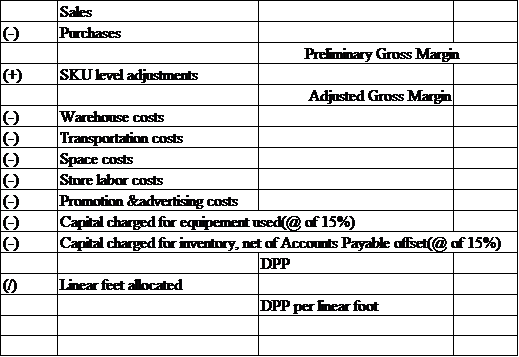Substitute: Here, Sale is$15,600,000, space cost is $2,400,000, advertising promotion is$300,000, refrigeration freezer Equipment is $1,000,000, dry product equipment is$1,000,000, and the rate of capital charged is 15%.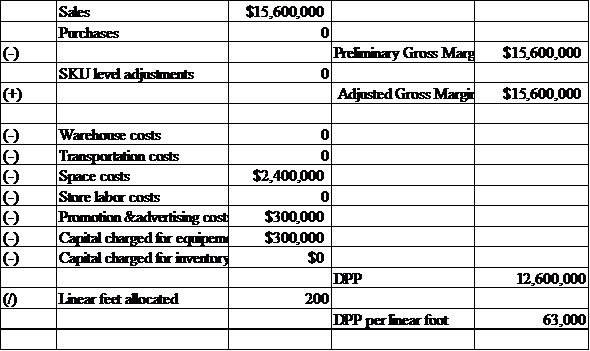Working notes:
Calculation of capital charge for equipment:
Given:Dry product equipment \$1,000,000Calculate the weekly profit impact to Flowers Bakery of the proposed change in delivery to Store #5.
Free
Essay

Calculate the weekly profit impact to Flowers Bakery of the proposed change in delivery to Store #5:
The profit is calculated through cost sales method, which compares cost to corresponding revenue. The profit is calculated as follows: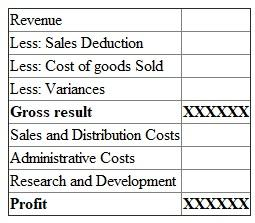By extracting the information: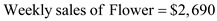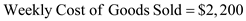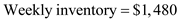Calculate the weekly profit: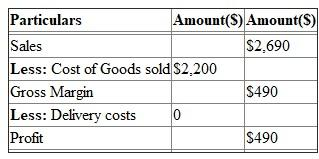Calculate the weekly profit impact to Store #5 of the proposal from Flowers.
EssayCalculate the annual DPP per "linear foot," after the switch in delivery mode by Flowers for each of the 12 bread SKUs ( day old is the 12 th SKU ).